# Stochastic approximation

(diff) ← Older revision | Latest revision (diff) | Newer revision → (diff)

A method for solving a class of problems of statistical estimation, in which the new value of the estimator is a modification of an existing estimator, based on new information. The first procedure of stochastic approximation was proposed in 1951 by H. Robbins and S. Monro.

Let every measurement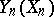of a function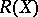,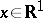, at a point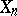contain a random error with mean zero. The Robbins–Monro procedure of stochastic approximation for finding a root of the equation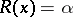takes the form(1)

If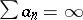,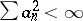, ifis, for example, an increasing function, ifincreases no faster than a linear function, and if the random errors are independent, thentends to a rootof the equationwith probability 1 and in the quadratic mean (see , ). It is clear from (1) that the process of stochastic approximation is recursive, i.e. a new value of the estimator can be obtained without recourse to the old measurement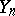, and is convenient in cases where the moment at which the estimator is to be represented is not known in advance. The estimator is formed continuously on the basis of observations relating to a given moment. These characteristics also pertain to stochastic approximation with recursive filters, and explain the popularity of stochastic approximation in theoretical and practical applications. The procedure (1) can be directly generalized to the multi-dimensional case.

Another procedure of stochastic approximation, used in finding a maximum point of a regression function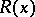, is attributed to J. Kiefer and J. Wolfowitz. Letbe an observation at the point. The Kiefer–Wolfowitz procedure then takes the form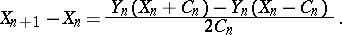(2)

It has been proved thatconverges to a maximum point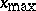of the functionif, for example,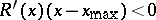when, if the regression function and the variance of the random errors do not increase too rapidly when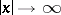, and if the conditions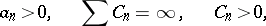are fulfilled. The Kiefer–Wolfowitz procedure of stochastic approximation also permits a multi-dimensional generalization: instead of the right-hand side in (2), an approximate value of the gradient of the function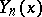has to be substituted.

Procedures of stochastic approximation can naturally be generalized to a continuous observation process. For example, if an observation process is disturbed by a Gaussian white noise, then the analogue of (1) takes the form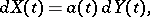where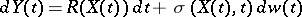is the differential of the process under observation andis a Wiener process. The conditions of convergence of continuous processes are analogous to those mentioned above for discrete time (see ). The basic instrument for proving the convergence of procedures of stochastic approximation is the theorem on the convergence of non-negative supermartingales (see Martingale).

The limit behaviour for an appropriate normalization of the difference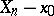whenhas been studied. In (1), letand let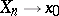almost certainly when. Given certain restrictions, foremost among which are the requirements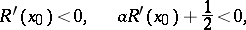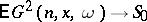where,, the asymptotic normality of the variable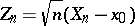with parameters 0,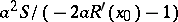has been proved. The least variance of the limit distribution is obtained for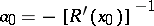. This choice ofis impossible, since the functionand its derivative are unknown values to be observed. However, in a number of works, adaptive procedures have been constructed in which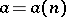depends on the observations and approximates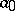when. These procedures possess properties that are asymptotically optimal in the sense of the asymptotic variance.

The results of asymptotic normality are also known in the multi-dimensional case. Let all roots of the matrixhave negative real parts (is the identity matrix), let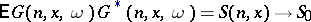when, letandalmost certainly, and let certain other not too-restrictive conditions be fulfilled. Then the vectoris asymptotically normal with mean zero and with covariance matrix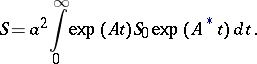The above result for an asymptotically-optimal Robbins–Monro procedure can also be generalized to the multi-dimensional case. It has been proved that the random processconverges to a Gaussian Markov process in a logarithmic scale. Given certain conditions, the convergence of the moments of the random variableto the moments of the limit law has been proved.

Stochastic approximation-type procedures are convenient in non-parametric situations, since they can be used when a priori information on the regression function is scarce. However, they are also used in estimating the parameterof the density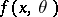through independent observationswith this density. Given certain restrictions, the recursive procedure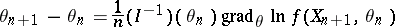(is the Fisher information matrix of the density) is a consistent and asymptotically-efficient recursive estimator of the parameter. The same process is also possible in the case of continuous time.

The behaviour of procedures of stochastic approximation has been studied in the case where the regression function has several zeros (several extremal points), and for different modifications and generalizations of procedures of stochastic approximation.

How to Cite This Entry:
Stochastic approximation. Encyclopedia of Mathematics. URL: http://encyclopediaofmath.org/index.php?title=Stochastic_approximation&oldid=17572
This article was adapted from an original article by R.Z. Khas'minskii (originator), which appeared in Encyclopedia of Mathematics - ISBN 1402006098. See original article